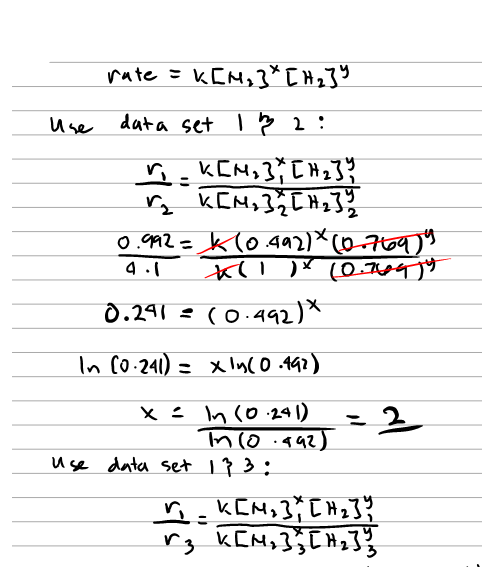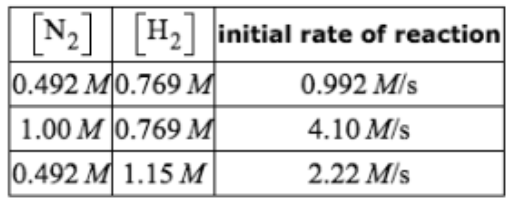# Problem: Some measurements of the initial rate of a certain reaction are given in the table below. Use this information to write a rate law for this reaction, and calculate the value of the rate constant k. Round your value for the rate constant to 2 significant digits. Also be sure your answer has the correct unit symbol.

🤓 Based on our data, we think this question is relevant for Professor Nagan's class at SUNY.

###### FREE Expert Solution###### Problem Details

Some measurements of the initial rate of a certain reaction are given in the table below.Use this information to write a rate law for this reaction, and calculate the value of the rate constant k.

Round your value for the rate constant to 2 significant digits. Also be sure your answer has the correct unit symbol.

What scientific concept do you need to know in order to solve this problem?

Our tutors have indicated that to solve this problem you will need to apply the Rate Law concept. You can view video lessons to learn Rate Law. Or if you need more Rate Law practice, you can also practice Rate Law practice problems.

What is the difficulty of this problem?

Our tutors rated the difficulty ofSome measurements of the initial rate of a certain reaction ...as medium difficulty.

How long does this problem take to solve?

Our expert Chemistry tutor, Sabrina took 10 minutes and 58 seconds to solve this problem. You can follow their steps in the video explanation above.

What professor is this problem relevant for?

Based on our data, we think this problem is relevant for Professor Nagan's class at SUNY.